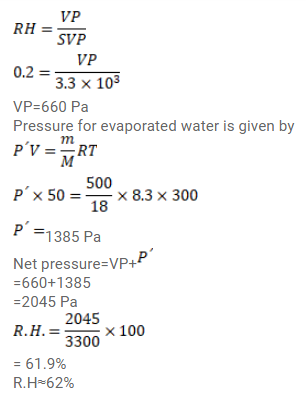# Use R=8.3 J/mol-K wherever required. The temperature and relative humidity are 300 KQuestion:

Use $\mathrm{R}=8.3 \mathrm{~J} / \mathrm{mol}-\mathrm{K}$ wherever required.

The temperature and relative humidity are $300 \mathrm{~K}$ and $20 \%$ in a room of volume $50 \mathrm{~m}^{3}$. The floor is washed with water, $500 \mathrm{~g}$ of water sticking on the floor. Assuming no communication with the surrounding, find the relative humidity when the floor dries. The changes in temperature and pressure may be neglected. Saturation vapour pressure at $300 \mathrm{~K}=3.3 \mathrm{kPa}$.

Solution: